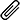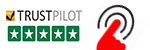High Quality, Fast Delivery, Plagiarism Free - Just in 3 Steps

## Upload Questions Details and Instructions:Select Assignment Files

## Get Assignment Help by Experts

### Plagiarism-Free, Every Time

All of our assignment solution delivers with a dedicated plagiarism report

### Excellent Subject Matter Expert

Get Supreme Assignment Help by highly Skilled Writers

### 100% Satisfaction Guaranteed

Score Higher or Your Money Back# MP122 Assignment Answers on Analytical and Statistical Techniques

## MP122 Question and Answers on Analytical and Statistical Techniques

Get a step closer to achieving an A+ grade with  Computer Science Assignment Help from our website’s certified CS Programming expert. Take your Assignment Answers on MP122 Analytical and Statistical Techniques. Casestudyhelp.com has experienced writers who provide you with 100% Plagiarism-free assignments that suit your budget. We deliver quality subject-specific assistance on more than 100+ subjects.### Question 1

(A) 1. A model can suffer from Omitted Variable Bias when one or more relevant variables gets missed and not included in the model. A variable can get considered as relevant when it is correlated with the dependent variable of the model. If this happens then the computed OLS estimators will also be biased.

2. A model is made up of two types of variables, dependent and independent variables. The issue of multicollinearity exists in the model when the independent variables in the model are correlated to each other. It becomes important to ensure that independent variables are uncorrelated in order to ensure high statistical significance of each independent variable in the model.

3. The presence of multicollinearity can lead to increase in the size of the standard error of the coefficients of the model. The increase in the size of the standard error can lead to occurrence of type 2 error because the researcher may end up accepting the false null hypothesis that the coefficient is equal to zero. Multicollinearity can also lead to type 1 error because the presence of multicollinearity reduces the overall significance of the model. The researcher may end up rejecting the true null hypothesis regarding the significance of coefficient of the model.

(B) Linear regression is an estimation of linear relationship between two or more variables. A linear equation is fitted into the observed data. This line consists of one dependent variable and one or more than one independent variables. In models, wherein there is only one independent variable it is regarded as simple linear regression and when there are more than one independent variables it is regarded as multiple linear regression.

According to the concept of regression fallacy, the results obtained or the relationship established is just due to the corrective actions .and no abnormalities. The concept of fallacy ignores the natural fluctuations that may also occur in variables over time.

1. R squared statistic explains the change caused in the dependent variable with the collective change in the independent variables included in the model. A higher R squared value indicates that the independent variables are all together able to explain significant proportion of change in the dependent variable.

A model is a good fit when it is able to fir the sample data provided. Both Standard Error of Regression and R squared statistic explains the goodness of fit of the model. However, there is a slight difference between these two statistics. SER explains the exact distance between the regression line and data points. Whereas, the R squared value explains the relative change caused in the dependent variable due to the collective change in the independent variable.

1. Dummy variables are quantitative variables but are included in numeric form in the model. The dummy variables are however in categorical in nature but are added in numerical form in the model.
1. The non-linear regression model can be fitted into the sample data in order to model the law of diminishing returns to scale.

### Question 2

1. The second model is better than the first model because the value of the adjusted model is greater in the second model as compared to the first model which means that addition of the variables is adding value to the model in the second case. Also, the value of the R squared statistic is also greater in the second case which means that the independent variables are able to explain greater amount of change in the dependent variable in the second model as compared to the first model.
1. The signs of both the regression coefficient are negative, that is the increase in the Student Teacher ratio will decrease the Test score. Also, the increase in the percentage of the English learners will also decrease the test score. However, this not the expected outcome. The researcher expected that the inclusion of the English learners will increase the test scores.
1. H0: There does not exist significant relationship between English learners and the test scores
1. HA: There exist significant relationship between English learners and the test scores.

We're here to instantly help! Get best assignment questions and answers help 24/7 and Earn better grades with homework